Calculus Volume 3

# Chapter 7

### Checkpoint

7.1
1. Nonlinear
2. Linear, nonhomogeneous
7.4

Linearly independent

7.5

$y ( x ) = c 1 e 3 x + c 2 x e 3 x y ( x ) = c 1 e 3 x + c 2 x e 3 x$

7.6
1. $y(x)=ex(c1cos3x+c2sin3x)y(x)=ex(c1cos3x+c2sin3x)$
2. $y(x)=c1e−7x+c2xe−7xy(x)=c1e−7x+c2xe−7x$
7.7

$y ( x ) = − e −2 x + e 5 x y ( x ) = − e −2 x + e 5 x$

7.8

$y(x)=ex(2cos3x−sin3x)y(x)=ex(2cos3x−sin3x)$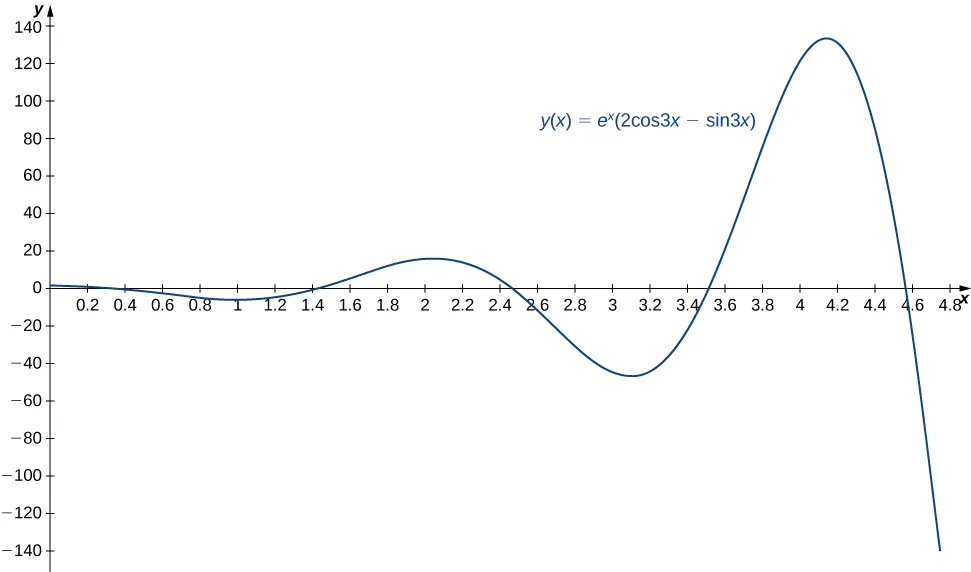7.9

$y(t)=te−7ty(t)=te−7t$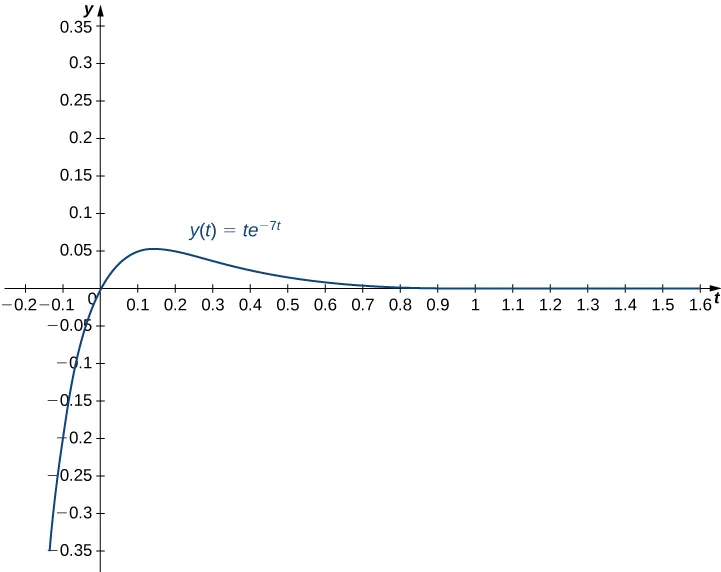At time $t=0.3,t=0.3,$ $y(0.3)=0.3e(−7*0.3)=0.3e−2.1≈0.0367.y(0.3)=0.3e(−7*0.3)=0.3e−2.1≈0.0367.$ The mass is 0.0367 ft below equilibrium. At time $t=0.1,t=0.1,$ $y′(0.1)=0.3e−0.7≈0.1490.y′(0.1)=0.3e−0.7≈0.1490.$ The mass is moving downward at a speed of 0.1490 ft/sec.

7.10

$y ( x ) = c 1 e − x + c 2 e 4 x − 2 y ( x ) = c 1 e − x + c 2 e 4 x − 2$

7.11

$y ( t ) = c 1 e 2 t + c 2 t e 2 t + sin t + cos t y ( t ) = c 1 e 2 t + c 2 t e 2 t + sin t + cos t$

7.12
1. $y(x)=c1e4x+c2ex−xexy(x)=c1e4x+c2ex−xex$
2. $y(t)=c1e−3t+c2e2t−5cos2t+sin2ty(t)=c1e−3t+c2e2t−5cos2t+sin2t$
7.13

$z1=3x+311x2,z1=3x+311x2,$ $z2=2x+211xz2=2x+211x$

7.14
1. $y(x)=c1cosx+c2sinx+cosxln|cosx|+xsinxy(x)=c1cosx+c2sinx+cosxln|cosx|+xsinx$
2. $x(t)=c1et+c2tet+tetln|t|x(t)=c1et+c2tet+tetln|t|$
7.15

$x(t)=0.1cos(14t)x(t)=0.1cos(14t)$ (in meters); frequency is $142π142π$ Hz.

7.16

$x(t)=17sin(4t+0.245),x(t)=17sin(4t+0.245),$ $frequency=42π≈0.637,frequency=42π≈0.637,$ $A=17A=17$

7.17

$x ( t ) = 0.6 e −2 t − 0.2 e −6 t x ( t ) = 0.6 e −2 t − 0.2 e −6 t$

7.18

$x ( t ) = 1 2 e −8 t + 4 t e −8 t x ( t ) = 1 2 e −8 t + 4 t e −8 t$

7.19

$x ( t ) = −0.24 e −2 t cos ( 4 t ) − 0.12 e −2 t sin ( 4 t ) x ( t ) = −0.24 e −2 t cos ( 4 t ) − 0.12 e −2 t sin ( 4 t )$

7.20

$x(t)=−12cos(4t)+94sin(4t)+12e−2tcos(4t)−2e−2tsin(4t)x(t)=−12cos(4t)+94sin(4t)+12e−2tcos(4t)−2e−2tsin(4t)$
$Transient solution:12e−2tcos(4t)−2e−2tsin(4t)Transient solution:12e−2tcos(4t)−2e−2tsin(4t)$
$Steady-state solution:−12cos(4t)+94sin(4t)Steady-state solution:−12cos(4t)+94sin(4t)$

7.21

$q ( t ) = −25 e − t cos ( 3 t ) − 7 e − t sin ( 3 t ) + 25 q ( t ) = −25 e − t cos ( 3 t ) − 7 e − t sin ( 3 t ) + 25$

7.22
1. $y(x)=a0∑n=0∞(−1)nn!x2n=a0e−x2y(x)=a0∑n=0∞(−1)nn!x2n=a0e−x2$
2. $y(x)=a0(x+1)3y(x)=a0(x+1)3$

### Section 7.1 Exercises

1.

linear, homogenous

3.

nonlinear

5.

linear, homogeneous

11.

$y = c 1 e 5 x + c 2 e −2 x y = c 1 e 5 x + c 2 e −2 x$

13.

$y = c 1 e −2 x + c 2 x e −2 x y = c 1 e −2 x + c 2 x e −2 x$

15.

$y = c 1 e 5 x / 2 + c 2 e − x y = c 1 e 5 x / 2 + c 2 e − x$

17.

$y = e − x / 2 ( c 1 cos 3 x 2 + c 2 sin 3 x 2 ) y = e − x / 2 ( c 1 cos 3 x 2 + c 2 sin 3 x 2 )$

19.

$y = c 1 e −11 x + c 2 e 11 x y = c 1 e −11 x + c 2 e 11 x$

21.

$y = c 1 cos 9 x + c 2 sin 9 x y = c 1 cos 9 x + c 2 sin 9 x$

23.

$y = c 1 + c 2 x y = c 1 + c 2 x$

25.

$y = c 1 e ( ( 1 + 22 ) / 3 ) x + c 2 e ( ( 1 − 22 ) / 3 ) x y = c 1 e ( ( 1 + 22 ) / 3 ) x + c 2 e ( ( 1 − 22 ) / 3 ) x$

27.

$y = c 1 e − x / 6 + c 2 x e − x / 6 y = c 1 e − x / 6 + c 2 x e − x / 6$

29.

$y = c 1 + c 2 e 9 x y = c 1 + c 2 e 9 x$

31.

$y = −2 e −2 x + 2 e −3 x y = −2 e −2 x + 2 e −3 x$

33.

$y = 3 cos ( 2 x ) + 5 sin ( 2 x ) y = 3 cos ( 2 x ) + 5 sin ( 2 x )$

35.

$y = − e 6 x + 2 e −5 x y = − e 6 x + 2 e −5 x$

37.

$y = 2 e − x / 5 + 7 5 x e − x / 5 y = 2 e − x / 5 + 7 5 x e − x / 5$

39.

$y = ( 2 e 6 − e −7 ) e 6 x − ( 2 e 6 − e −7 ) e −7 x y = ( 2 e 6 − e −7 ) e 6 x − ( 2 e 6 − e −7 ) e −7 x$

41.

No solutions exist.

43.

$y = 2 e 2 x − 2 e 2 + 1 e 2 x e 2 x y = 2 e 2 x − 2 e 2 + 1 e 2 x e 2 x$

45.

$y = 4 cos 3 x + c 2 sin 3 x , infinitely many solutions y = 4 cos 3 x + c 2 sin 3 x , infinitely many solutions$

47.

$5 y ″ + 19 y ′ − 4 y = 0 5 y ″ + 19 y ′ − 4 y = 0$

49.

a. $y=3cos(8x)+2sin(8x)y=3cos(8x)+2sin(8x)$
b.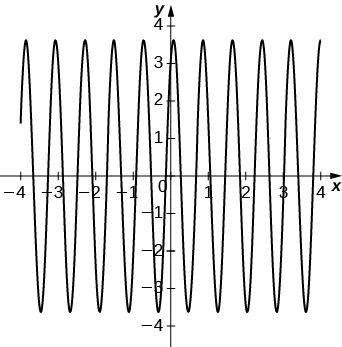51.

a. $y=e(−5/2)x[−2cos(352x)+43535sin(352x)]y=e(−5/2)x[−2cos(352x)+43535sin(352x)]$
b.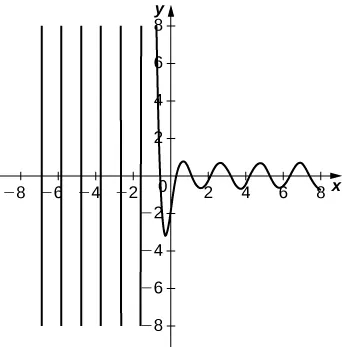### Section 7.2 Exercises

55.

$y = c 1 e −4 x / 3 + c 2 e x − 2 y = c 1 e −4 x / 3 + c 2 e x − 2$

57.

$y = c 1 cos 4 x + c 2 sin 4 x + 1 20 e −2 x y = c 1 cos 4 x + c 2 sin 4 x + 1 20 e −2 x$

59.

$y = c 1 e 2 x + c 2 x e 2 x + 2 x 2 + 5 x + 4 y = c 1 e 2 x + c 2 x e 2 x + 2 x 2 + 5 x + 4$

61.

$y = c 1 e − x + c 2 x e − x + 1 2 sin x − 1 2 cos x y = c 1 e − x + c 2 x e − x + 1 2 sin x − 1 2 cos x$

63.

$y = c 1 cos x + c 2 sin x − 1 3 x cos 2 x − 5 9 sin 2 x y = c 1 cos x + c 2 sin x − 1 3 x cos 2 x − 5 9 sin 2 x$

65.

$y = c 1 e −5 x + c 2 x e −5 x + 1 6 x 3 e −5 x + 4 25 y = c 1 e −5 x + c 2 x e −5 x + 1 6 x 3 e −5 x + 4 25$

67.

a. $yp(x)=Ax2+Bx+Cyp(x)=Ax2+Bx+C$
b. $yp(x)=−13x2+43x−359yp(x)=−13x2+43x−359$

69.

a. $yp(x)=(Ax2+Bx+C)e−xyp(x)=(Ax2+Bx+C)e−x$
b. $yp(x)=(14x2−58x−3332)e−xyp(x)=(14x2−58x−3332)e−x$

71.

a. $yp(x)=(Ax2+Bx+C)excosxyp(x)=(Ax2+Bx+C)excosx$ $+(Dx2+Ex+F)exsinx+(Dx2+Ex+F)exsinx$
b. $yp(x)=(−110x2−1125x−27250)excosxyp(x)=(−110x2−1125x−27250)excosx$ $+(−310x2+225x+39250)exsinx+(−310x2+225x+39250)exsinx$

73.

$y = c 1 + c 2 e −2 x + 1 15 e 3 x y = c 1 + c 2 e −2 x + 1 15 e 3 x$

75.

$y = c 1 e 2 x + c 2 e −4 x + x e 2 x y = c 1 e 2 x + c 2 e −4 x + x e 2 x$

77.

$y = c 1 e 3 x + c 2 e −3 x − 8 x 9 y = c 1 e 3 x + c 2 e −3 x − 8 x 9$

79.

$y = c 1 cos 2 x + c 2 sin 2 x − 3 2 x cos 2 x + 3 4 sin 2 x ln ( sin 2 x ) y = c 1 cos 2 x + c 2 sin 2 x − 3 2 x cos 2 x + 3 4 sin 2 x ln ( sin 2 x )$

81.

$y = − 347 343 + 4 343 e 7 x + 2 7 x 2 e 7 x − 4 49 x e 7 x y = − 347 343 + 4 343 e 7 x + 2 7 x 2 e 7 x − 4 49 x e 7 x$

83.

$y = − 57 25 + 3 25 e 5 x + 1 5 x e 5 x + 4 25 e −5 x y = − 57 25 + 3 25 e 5 x + 1 5 x e 5 x + 4 25 e −5 x$

85.

$y p = 1 2 + 10 3 x 2 ln x y p = 1 2 + 10 3 x 2 ln x$

### Section 7.3 Exercises

87.

$x″+16x=0,x″+16x=0,$ $x(t)=16cos(4t)−2sin(4t),x(t)=16cos(4t)−2sin(4t),$ period $=π2sec,=π2sec,$ frequency $=2πHz=2πHz$

89.

$x″+196x=0,x″+196x=0,$ $x(t)=0.15cos(14t),x(t)=0.15cos(14t),$ period $=π7sec,=π7sec,$ frequency $=7πHz=7πHz$

91.

a. $x(t)=5sin(2t)x(t)=5sin(2t)$
b. period $=πsec,=πsec,$ frequency $=1πHz=1πHz$
c.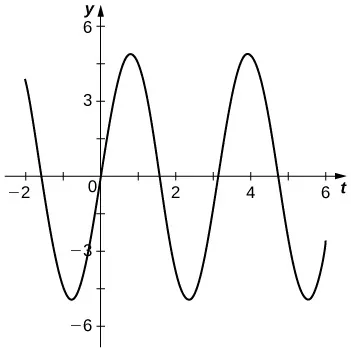d. $t=π2sect=π2sec$

93.

a. $x(t)=e−t/5(20cos(3t)+15sin(3t))x(t)=e−t/5(20cos(3t)+15sin(3t))$
b. underdamped

95.

a. $x(t)=5e−4t+10te−4tx(t)=5e−4t+10te−4t$
b. critically damped

97.

$x(π)=7e−π/46x(π)=7e−π/46$ ft below

99.

$x ( t ) = 32 9 sin ( 4 t ) + cos ( 128 t ) − 16 9 2 sin ( 128 t ) x ( t ) = 32 9 sin ( 4 t ) + cos ( 128 t ) − 16 9 2 sin ( 128 t )$

101.

$q ( t ) = e −6 t ( 0.051 cos ( 8 t ) + 0.03825 sin ( 8 t ) ) − 1 20 cos ( 10 t ) q ( t ) = e −6 t ( 0.051 cos ( 8 t ) + 0.03825 sin ( 8 t ) ) − 1 20 cos ( 10 t )$

103.

$q ( t ) = e −10 t ( −32 t − 5 ) + 5 , I ( t ) = 2 e −10 t ( 160 t + 9 ) q ( t ) = e −10 t ( −32 t − 5 ) + 5 , I ( t ) = 2 e −10 t ( 160 t + 9 )$

### Section 7.4 Exercises

105.

$y = c 0 + 5 c 1 ∑ n = 1 ∞ ( − x / 5 ) n n ! = c 0 + 5 c 1 e − x / 5 y = c 0 + 5 c 1 ∑ n = 1 ∞ ( − x / 5 ) n n ! = c 0 + 5 c 1 e − x / 5$

107.

$y = c 0 ∑ n = 0 ∞ ( x ) 2 n ( 2 n ) ! + c 1 ∑ n = 0 ∞ ( x ) 2 n + 1 ( 2 n + 1 ) ! y = c 0 ∑ n = 0 ∞ ( x ) 2 n ( 2 n ) ! + c 1 ∑ n = 0 ∞ ( x ) 2 n + 1 ( 2 n + 1 ) !$

109.

$y = c 0 ∑ n = 0 ∞ x 2 n n ! = c 0 e x 2 y = c 0 ∑ n = 0 ∞ x 2 n n ! = c 0 e x 2$

111.

$y = c 0 ∑ n = 0 ∞ x 2 n 2 n n ! + c 1 ∑ n = 0 ∞ x 2 n + 1 1 ⋅ 3 ⋅ 5 ⋅ 7 ⋯ ( 2 n + 1 ) y = c 0 ∑ n = 0 ∞ x 2 n 2 n n ! + c 1 ∑ n = 0 ∞ x 2 n + 1 1 ⋅ 3 ⋅ 5 ⋅ 7 ⋯ ( 2 n + 1 )$

113.

$y = c 1 x 3 + c 2 x y = c 1 x 3 + c 2 x$

115.

$y = 1 − 3 x + 2 x 3 3 ! − 12 x 4 4 ! + 16 x 6 6 ! − 120 x 7 7 ! + ⋯ y = 1 − 3 x + 2 x 3 3 ! − 12 x 4 4 ! + 16 x 6 6 ! − 120 x 7 7 ! + ⋯$

### Review Exercises

117.

True

119.

False

121.

second order, linear, homogeneous, $λ2−2=0λ2−2=0$

123.

first order, nonlinear, nonhomogeneous

125.

$y = c 1 sin ( 3 x ) + c 2 cos ( 3 x ) y = c 1 sin ( 3 x ) + c 2 cos ( 3 x )$

127.

$y = c 1 e x sin ( 3 x ) + c 2 e x cos ( 3 x ) + 2 5 x + 2 25 y = c 1 e x sin ( 3 x ) + c 2 e x cos ( 3 x ) + 2 5 x + 2 25$

129.

$y = c 1 e − x + c 2 e −4 x + x 4 + e 2 x 18 − 5 16 y = c 1 e − x + c 2 e −4 x + x 4 + e 2 x 18 − 5 16$

131.

$y = c 1 e ( −3 / 2 ) x + c 2 x e ( −3 / 2 ) x + 4 9 x 2 + 4 27 x − 16 27 y = c 1 e ( −3 / 2 ) x + c 2 x e ( −3 / 2 ) x + 4 9 x 2 + 4 27 x − 16 27$

133.

$y = e −2 x sin ( 2 x ) y = e −2 x sin ( 2 x )$

135.

$y = e 1 − x e 4 − 1 ( e 4 x − 1 ) y = e 1 − x e 4 − 1 ( e 4 x − 1 )$

137.

$θ ( t ) = θ 0 cos ( g l t ) θ ( t ) = θ 0 cos ( g l t )$

141.

$b = a b = a$

Order a print copy

As an Amazon Associate we earn from qualifying purchases.